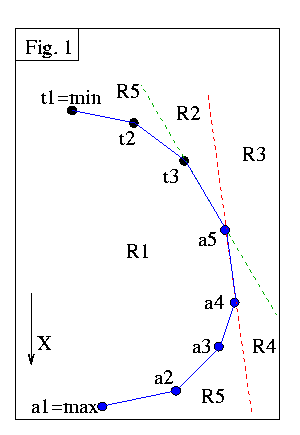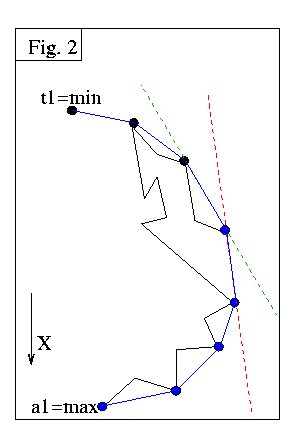McCallum & Avis 1979First Correct Algorithm

McCallum and Avis are credited with the first correct convex hull algorithm . They show how to compute the left Hull, i.e. the hull between Xmax and Xmin which has the polygonal region to its left.

The authors use two stacks. Stack A contains all the vertices tentatively accepted as convex hull points. Upon termination, this stack contains the hull, with vertices in decreasing order from bottom to top. Stack T contains points which have been rejected as hull vertices, but which are on the hull of the input seen so far. Xmin is always at the bottom of T, and in the end is the only vertex in T. The authors state that stack T is essential for the rejection of certain types of vertices. This is not true, as you may see in following sections, where only one stack is used.

Fig.1 is used by the authors to explain the algorithm. The red line is defined by the top two vertices of A. The green line is defined by the top of each stack. Region 1 is the hull of all points in both stacks, plus all points to the left of the line (Xmax,Xmin). Regions 2,3,4 are obvious from the figure. Region 5 is the complement of regions 1-4.

The authors state that in stack T the vertices are in decreasing order from bottom to top, with the exception of Xmin, which is always at the bottom. Thus we could imagine that the situation of Fig.1 has occured from a polygonal chain such as the one shown in black in Fig.2: (expand browser horizontally to see figures side by side).All the points except Xmin have been checked. Blue points are in A because they are on the hull, so far. Black points are in T, because they are on the hull of points seen so far, but can't be on the final hull, due to the Jordan curve theorem. All other vertices are inside both hulls.

The authors consider examining the next vertex K on the polygon:

If K is in R1, reject it.

If K is in R2, add it to A, and backtrack T, since some vertices no longer belong there.

If K is in R3, backtrack both stacks.

If K is in R4, remove the top of A and place it in T, then backtrack A.

Note that K cannot be in R5. As you can see from Fig.1, testing a point to determine which region it is in requires a few comparisons to lines. Backtracking is similar to the Sklansky scan.

Finally, a note for anyone interested in implementing this algorithm: On p.204, in "Procedure halfhull" which performs the above operations, it seems that on the 18th line from the bottom the word "right" should be replaced by "left" in order for A to backtrack correctly.

previous : Shamos 1975.          next : Kim 1980.